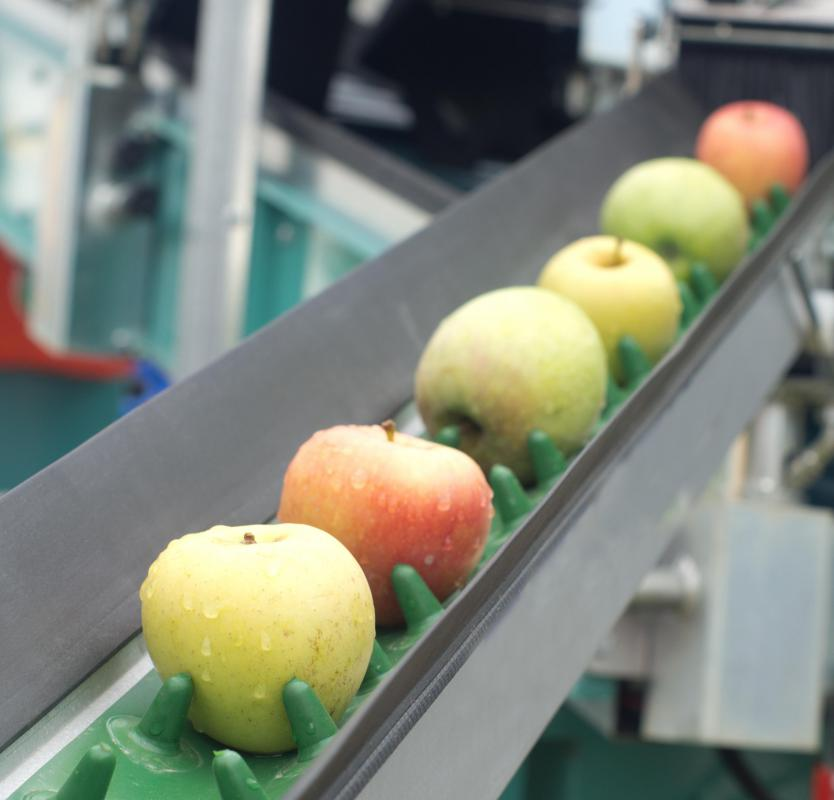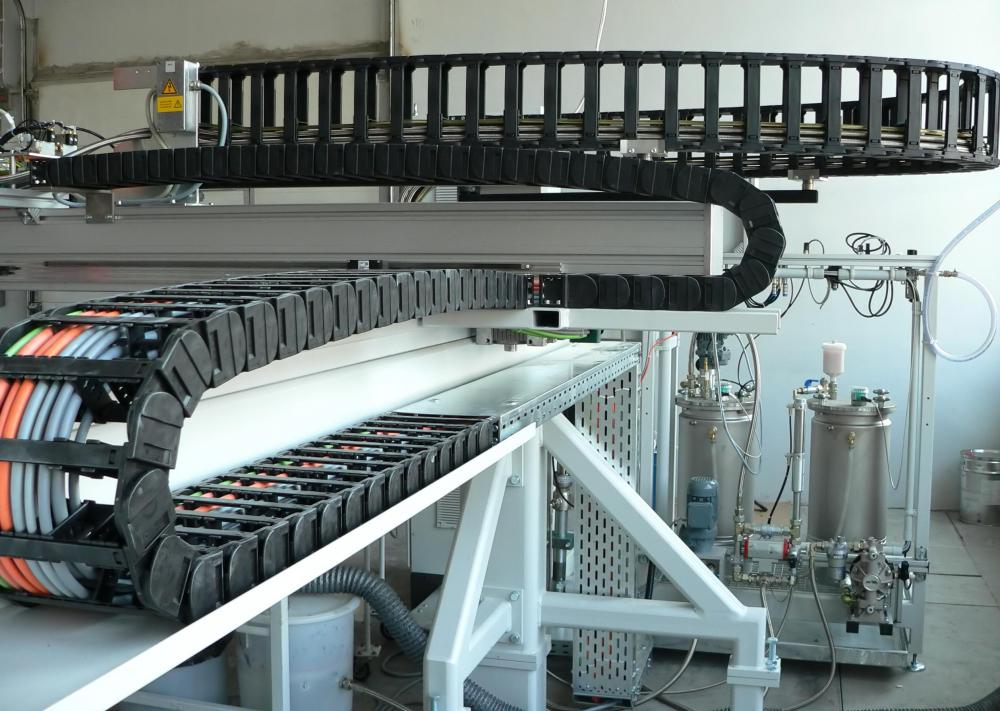# How Do I Calculate Conveyor Belt Tension?

Lakshmi Sandhana

Getting the conveyor belt tension right is imperative for the system to work smoothly. The belt needs to conform to the crown of any pulley present and be slip resistant even under the most challenging external conditions. To calculate the conveyor belt tension accurately, start by collecting all the information that you need and enter it in a form. You need to calculate the maximum amount of tension that you can put on the belt system, which you can figure out by using several specific formulas.Accurate information must be gathered in order to calculate conveyor belt tension.

First, gather information on the working environment and any special conditions the system has to operate under, for example, the loading rate at tons per hour, the pulley diameter, and carry idlers used. Note the speed of the belt, width of the belt, and the vertical difference between the tail and head pulleys. Aside from the height, the length of the belt and drive data are also important to calculate conveyor belt tension. It's easy to calculate the operation tension, or TO, also called allowable working tension, once you have all the data on hand.A conveyor belt system itself needs to be "trained," meaning the belt must be adjusted in such a way that it does not run off track.

The first factor to calculate when figuring out conveyor belt tension is the tension needed to move the empty belt, or TC. You'll need to know the weight of the conveyor belt components, or CW, the belt length, or L, and the friction factor for normal empty belt operation, or F1. F1 is normally 0.035, and the formula for calculating TC is TC = F1 x L x CW or TC = 0.035 x L x CW.

The tension required to move the materials on the belt horizontally, or TL, is the next step in calculating conveyor belt tension. You'll need to know the material weight in pounds, or MW, and multiply it by the belt length and the typical friction factor needed to move a load horizontally, or F2. F2 is normally 0.04, and the formula for calculating TL is TL = MW x L x F2 or MW x L x 0.04.

TH is the tension needed to lift the load, and this is found my multiplying MW with the difference in elevation of the terminal pulleys, or H. The equation for TH is TH = MW x H. Once you have these three factors, you can calculate the effective belt tension, or TE. The formula is TE = TC + TL + TH. It's also necessary to factor in slack side tension, or TS, which is needed to prevent belt slippage. So, TS = D x TE, where D stands for the drive factor.

You can finally figure out the total operating tension, or TO, by knowing the belt width in inches, or W, TS, and TE. The formula for TO is TO = TE + TS/W. Over the life of the conveyor system, you may need to retension the belt. A contaminant could get between the pulley surface and the conveyor belt surface, and the relative coefficient of friction may be altered as result. Cleaning all surfaces usually takes care of the problem, but if this is impossible due to external conditions, then it may be necessary to increase the conveyor belt tension.Proper conveyor belt tension is important to the machine's function and efficiency.

## You might also Like